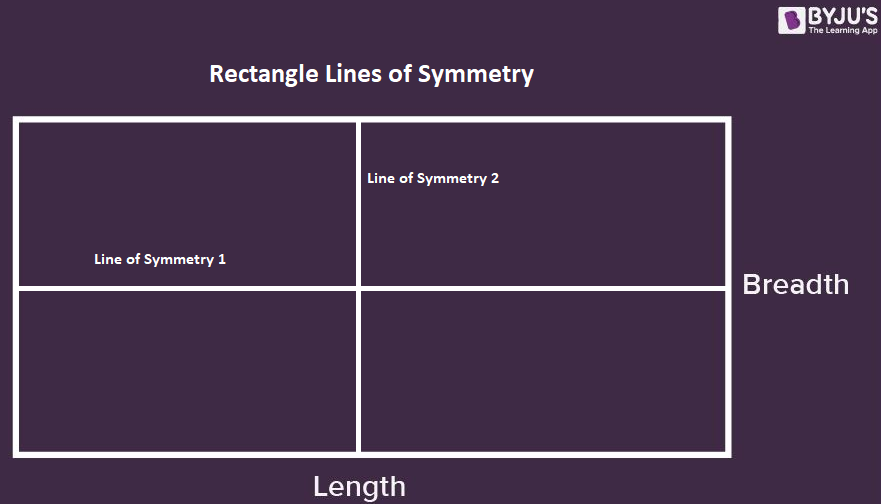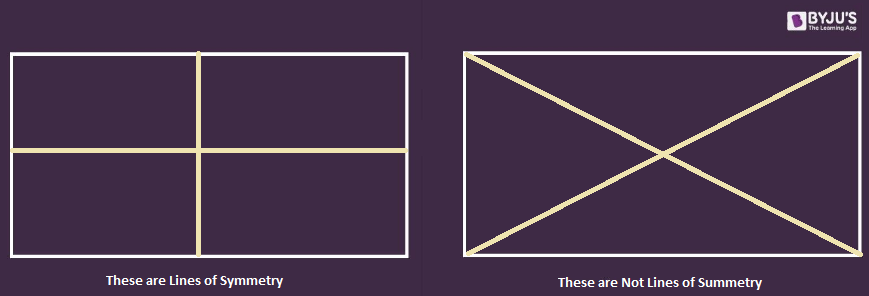# Lines of Symmetry in a Rectangle

A rectangle has 2 lines of symmetry which divides it into two identical parts. A shape can be different types of symmetry, such as linear symmetry, mirror symmetry, reflectional symmetry, and so on. A shape can be two or more lines of symmetry. To recall, a rectangle is one of the quadrilaterals whose two opposite sides are equal and parallelogram. Thus, a rectangle is also considered as a special parallelogram. For a rectangle, both the line and rotational symmetry exists which are discussed below.

## What is Lines of Symmetry in Rectangle?

There are 2 symmetry lines of a rectangle which are from its length and breadth. These two lines cut the rectangle in two similar halves which are mirror images of each other. If a rectangle is folded along its line of symmetry, it superimposes perfectly.

 Number of Symmetry Lines in a Rectangle = 2

Here are the two lines of symmetry in a rectangle:Lines Of Symmetry In A Rectangle

It should be noted that for a rectangle, the diagonals are not its lines of symmetry. This is because, if a rectangle cut along its diagonals will not superimpose perfectly as the sides will be of different measurement.### Rotational Symmetry of a Rectangle

A rectangle has an order of rotational symmetry of 2 at 180° and 360°. This means a rectangle can map onto itself 2 times during rotation of 360° i.e. at 180° and 360°. This is in contrast to a square which has rotational symmetry of 4 as it has 4 equal sides.

## Frequently Asked Questions on Lines Of Symmetry In A Rectangle

### What is a Rectangle?

A rectangle is a 4 sided polygon whose two opposite sides are equal and parallel. The angles of a rectangle are always 90 degrees and its diagonals are always equal.

### How Many Lines of Symmetry Does a Rectangle Have?

A rectangle has 2 lines of symmetry. The lines of symmetry in a rectangle cut its opposite sides into equal parts.

### Does a Rectangle Have Rotational Symmetry?

Yes, a rectangle has rotational symmetry. A rectangle has rotational symmetry of 180° (Order 2).

Quiz on Lines of symmetry in a rectangle